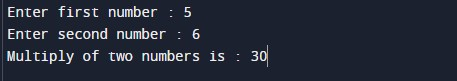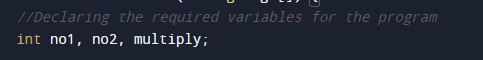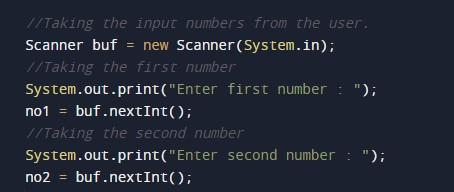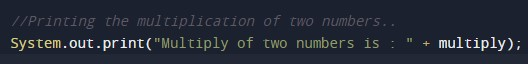# Java Program to Multiply two Numbers

In this tutorial you will learn about the Java Program to Multiply two Numbers and its application with practical example.

## Java Program to Multiply Two Numbers

In this tutorial, we will learn to create a Java program that will Multiply Two Numbers in Java programming.

## Prerequisites

Before starting with this tutorial, we assume that you are the best aware of the following Java programming topics:

• Operators in Java Programming.
• Basic Input and Output function in Java Programming.
• Basic Java Programming.
• Arithmetic operations in java programming.

## Program to Multiply Two Numbers:-

In today’s tutorial, we will create a program that will multiply the two numbers using the concepts of java programming. First, we will take the two numbers in input from the user. Then we will multiply the two numbers from the code. At last, we will print the output of the program.

With the help of this program, we can take input and Multiply Two Numbers.

## Output:-In the above program, we have first initialized the required variable.• no1 = it will hold the input number value from the user.
• no2 = it will hold the input number value from the user.
• Multiply = it will hold the sum value of the numbers.

Taking input numbers from the user.Calculating the multiply of two numbers.Printing the output.In this tutorial we have learn about the Java Program to Multiply two Numbers and its application with practical example. I hope you will like this tutorial.